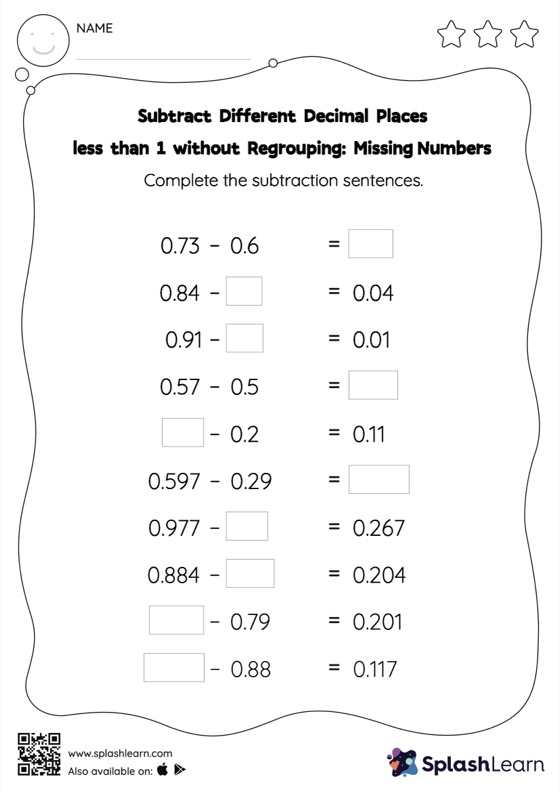# Subtract Different Decimal Places less than 1 without Regrouping: Missing Numbers Worksheet

Home > Subtract Different Decimal Places less than 1 without Regrouping: Missing NumbersWhile subtracting decimals, students use the relationship between addition and subtraction to find the missing number. They do not need to regroup numbers in subtract different decimal places less than 1 without regrouping worksheet. This worksheet is about practicing with the horizontal format in which numbers are written side by side. To develop flexibility with numbers and operations, students need to have enough practice in this format and not just rely on the vertical/column method.